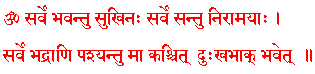# SAMSKRUTAM Studies

Welcome Guest!  | Login (internal use)
Home | Site Map | Sanskrit Editor | Help (?) |  »

# Grammar Tutorial ::: Euphonic Combination / व्यञ्जनसन्धि / vyaJnjanasandhi

DRAFT CONTENT, IN-PROGRESS.
व्यञ्जनसन्धि (vyaJnjanasandhi): In this chapter we will study about व्यञ्जनसन्धि (vyaJnjanasandhi).

Note: When two words are combined,
• the first words is called the पूर्वपद (puurvapada);
• the second word is called the परपद (parapada); and
• the combined word is called the एकपद (ekapada).

Study the following sentences.
पूर्वपद
परपद
एकपद
वर्णविभाग
varNavibhaaga
सन्धि
sandhi
Notes
1.दिक्
dik
अन्तः
antaH
दिगन्तः
digantaH
(द् इ क्) + (अ न् त् अः) = (द् इ ग् अ न् त् अः)
(d i k) + (a n t aH) = (d i g a n t aH)
क् + अ = ग्
k + a = ga
...
2.दिक्
dik
अन्तः
antaH
दिगन्तः
digantaH
(द् इ क्) + (अ न् त् अः) = (द् इ ग् अ न् त् अः)
(d i k) + (a n t aH) = (d i g a n t aH)
क् + अ = ग्
k + a = ga

Grammatical Rule
If the .... will take place.

In words 1 to 5 ... So, ... has taken place and ... has been substituted with....

Grammatical Rule
If the .... will take place.

In words 1 to 5 ... So, ... has taken place and ... has been substituted with....

Grammatical Rule
If the .... will take place.

In words 1 to 5 ... So, ... has taken place and ... has been substituted with....

Grammatical Rule
If the .... will take place.

In words 1 to 5 ... So, ... has taken place and ... has been substituted with....

Study the following sentences.
पूर्वपद
परपद
एकपद
वर्णविभाग
varNavibhaaga
सन्धि
sandhi
Notes
1.दिक्
dik
अन्तः
antaH
दिगन्तः
digantaH
(द् इ क्) + (अ न् त् अः) = (द् इ ग् अ न् त् अः)
(d i k) + (a n t aH) = (d i g a n t aH)
क् + अ = ग्
k + a = ga
...
2.दिक्
dik
अन्तः
antaH
दिगन्तः
digantaH
(द् इ क्) + (अ न् त् अः) = (द् इ ग् अ न् त् अः)
(d i k) + (a n t aH) = (d i g a n t aH)
क् + अ = ग्
k + a = ga

Grammatical Rule
If the .... will take place.

In words 1 to 5 ... So, ... has taken place and ... has been substituted with....

Grammatical Rule
If the .... will take place.

In words 1 to 5 ... So, ... has taken place and ... has been substituted with....

Grammatical Rule
If the .... will take place.

In words 1 to 5 ... So, ... has taken place and ... has been substituted with....

Grammatical Rule
If the .... will take place.

In words 1 to 5 ... So, ... has taken place and ... has been substituted with....Except where otherwise noted, this work by SAMSKRUTAM is licensed under a Creative Commons Attribution-ShareAlike 3.0 Unported License. You can copy, distribute, transmit, adapt, and make use of the work (including commercial); with attribution of this work to SAMSKRUTAM.